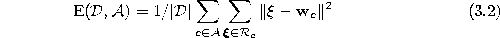Next: Entropy Maximization Up: Goals of Competitive Learning Previous: Goals of Competitive Learning

# Error Minimization

A frequent goal is the minimization of the expected quantization (or distortion) error. In the case of a continuous input signal distributionthis amounts to finding values for the reference vectorssuch that the erroris minimized (is the Voronoi region of unit c).

Correspondingly, in the case of a finite data setthe errorhas to be minimized withbeing the Voronoi set of the unit c.

A typical application where error minimization is important is vector quantization (Gray, 1984; Linde et al., 1980). In vector quantization data is transmitted over limited bandwidth communication channels by transmitting for each data vector only the index of the nearest reference vector. The set of reference vectors (which is called codebook in this context) is assumed to be known both to sender and receiver. Therefore, the receiver can use the transmitted indexes to retrieve the corresponding reference vector. There is an information loss in this case which is equal to the distance of current data vector and nearest reference vector. The expectation value of this error is described by equations (3.1) and (3.2). In particular if the data distribution is clustered (contains subregions of high probability density), dramatic compression rates can be achieved with vector quantization with relatively little distortion.

Bernd Fritzke
Sat Apr 5 18:17:58 MET DST 1997